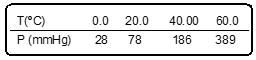Clutch Prep is now a part of Pearson
Ch.11 - Liquids, Solids & Intermolecular ForcesWorksheetSee all chapters

# Clausius-Clapeyron Equation

See all sections
Sections
Molecular Polarity
Intermolecular Forces
Intermolecular Forces and Physical Properties
Clausius-Clapeyron Equation
Phase Diagrams
Heating and Cooling Curves
Atomic, Ionic, and Molecular Solids
Crystalline Solids
Simple Cubic Unit Cell
Body Centered Cubic Unit Cell
Face Centered Cubic Unit Cell

The Clausius-Clapeyron Equation establishes a quantitative relationship between vapor pressure and temperature

###### Examining the Clausius-Clapeyron Equation

Concept #1: Understanding the Clasius-Clapeyron Equation

Vapor Pressure looks at the equilibrium established between vaporization and condensation. By using the Clasius-Clapeyron equation, the enthalpy of vaporization can be determined.

Concept #2: Linear Form of Clausius-Clapeyron Equation

Linear Form of Clausius-Clapeyron Equation used when given a plot of lnP vs inverse of Temperature.

Example #1: The vapor pressure of a substance is measured over a range of temperatures. A plot of the natural log of the vapor pressure vs the inverse of the temperatures (in Kelvin) produces a straight line with a slope of -2.79 x 103 K.

Find the enthalpy of vaporization of the substance.

Practice: Vapor pressure measurements at various temperature values are given below. Determine the molar heat of vaporization for cyclohexane.Concept #3: Two-Point Form of Clausius-Clapeyron Equation

Two-Point Form of Clausius-Clapeyron Equation used when 2 Temperatures and 2 Pressures are involved.

Example #2: The enthalpy of vaporization of water is 40.3 kJ/mol at its normal boiling point at 100ºC. What is the vapor pressure (mmHg) of water at 60ºC?

Practice: Benzene has a heat of vaporization of 30.72 kJ/mol and a normal boiling point of 80.1°C. At what temperature does benzene boil when the external pressure is 405 torr?